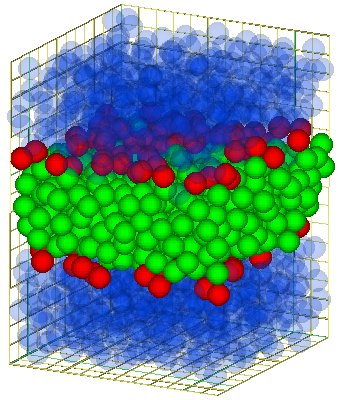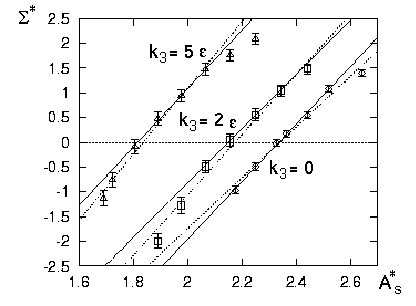## Stretching elasticity of model bilayersThe simplest form stretching elasticity is the Hookean law. By taking the derivative of the free energy with respect to the relative area change one obtain from the Hookean law that the surface tension is proportional to the surface area (at fixed number of molecules).

Since 1948 one has a formula to determine the stress tensor for molecular fluids interacting via two-body-potentials. Using this formula and a newly derived formula for three body-potentials (for details see [1,2]) we determined the stress tensor and calculated the surface tension out of this tensor at each timestep of our simulation.

For each model surfactant (ht2, HT2 at two different tail stiffnesses and H3(T4)2) a couple simulation at different areas per surfactant molecule has been performed. At all those simulation the over all volume, the overall density and the number of surfactant molecule were kept constant. By varying the width and height of the simulation box different compressions and stresses were put on the bilayers.

One finds that the surface tension increases linearly with the areas per surfactant molecule around the tensionless membrane (see the figure below). However, for larger stresses the behavior become sub-linear. This can be understood of one comparing the loss of entropy in case of a stretched and a compressed membrane.Surface tension of model bilayers against the area per molecule for the one chains surfactants ht2 and HT2. Please note that k3 is the tail-stiffness. k3=0 is equivalent to ht2-surfactants.

#### Stress Profile

Furthermore the stress profile across the bilayer has been investigated. It turns out that this profile is more complicated than expected. For details see .

#### Shear

Another aspect of the stress tensor is that its non-diagonal components are a measure for the shear moduli. However the values of this components vanish, apart from stastical noise. Hence there is non shear rigidity. This was expected as the bilayers are in fluid state, as shown before.

### Conclusion

It has been shown that the Hookean law applies to same systems with only 64 surfactants in a monolayer. Hence the gap between molecular description and the elasticity theory has been closed at this point.

#### Literature:

1. R. Goetz; PhD-thesis, Universität Potsdam 1997.
2. R. Goetz, R. Lipowsky; J. Chem. Phys. 108, (1998), 7397-7409
3. R. Goetz, R. Lipowsky; Phys. Rev. Letters 82, (1999), 221-224

Bei Fragen, Anregungen usw. schickt mir doch eine Mail.
Die Datenschutzerklärung finden sie hier. Das Impressum finden sie hier.